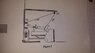# Broken leg & pulleys

stunner5000pt

## Homework Statement

assume that the total downward force exerted at A & B by the leg is 38N. find the weight W, attached on the lower puller

## The Attempt at a Solution

[/B]
i have written roman numerals to help determine which equation refers to which point

at point I
$$W = T$$

at point II
in the X direction
$$T \cos 25 + F_{1} = F_{A} cos \theta$$
in the y direction

$$T \sin 25 = F_{A} \sin \theta$$

at point III
in the X direction
$$0=T \cos 20 +T \cos 25$$

in the Y direction

$$T \sin 20 + T \sin 25$$

at point IV

in the X direction

$$T \cos 20 + T \cos 60 = 0$$

in the Y direction

$$-T \sin 20 - T\sin 60 = 0$$

And that the end of this, can we say that T = 38N?

Please let me know if all the equations are correct?
Thank you for your input and assistance!

#### Attachments

•leg question.jpg
23.6 KB · Views: 415

Homework Helper
Gold Member

## Homework Statement

assume that the total downward force exerted at A & B by the leg is 38N. find the weight W, attached on the lower puller

## The Attempt at a Solution

[/B]
i have written roman numerals to help determine which equation refers to which point

at point I
$$W = T$$

at point II
in the X direction
$$T \cos 25 + F_{1} = F_{A} cos \theta$$
in the y direction

$$T \sin 25 = F_{A} \sin \theta$$

at point III
in the X direction
$$0=T \cos 20 +T \cos 25$$

in the Y direction

$$T \sin 20 + T \sin 25$$

at point IV

in the X direction

$$T \cos 20 + T \cos 60 = 0$$

in the Y direction

$$-T \sin 20 - T\sin 60 = 0$$

And that the end of this, can we say that T = 38N?

Please let me know if all the equations are correct?
Thank you for your input and assistance!
Well I'm not sure of this problem but if the lower leg is horizontal and takes only an axial pulling force F1 , then draw the FBD of the pulley at the foot and sum vertical forces to solve for T and thence mg. F-a is given I think as a vert force of 38 N at A so I don't know why you are breaking it into components. You are also missing a tension force in your equation at that pulley.